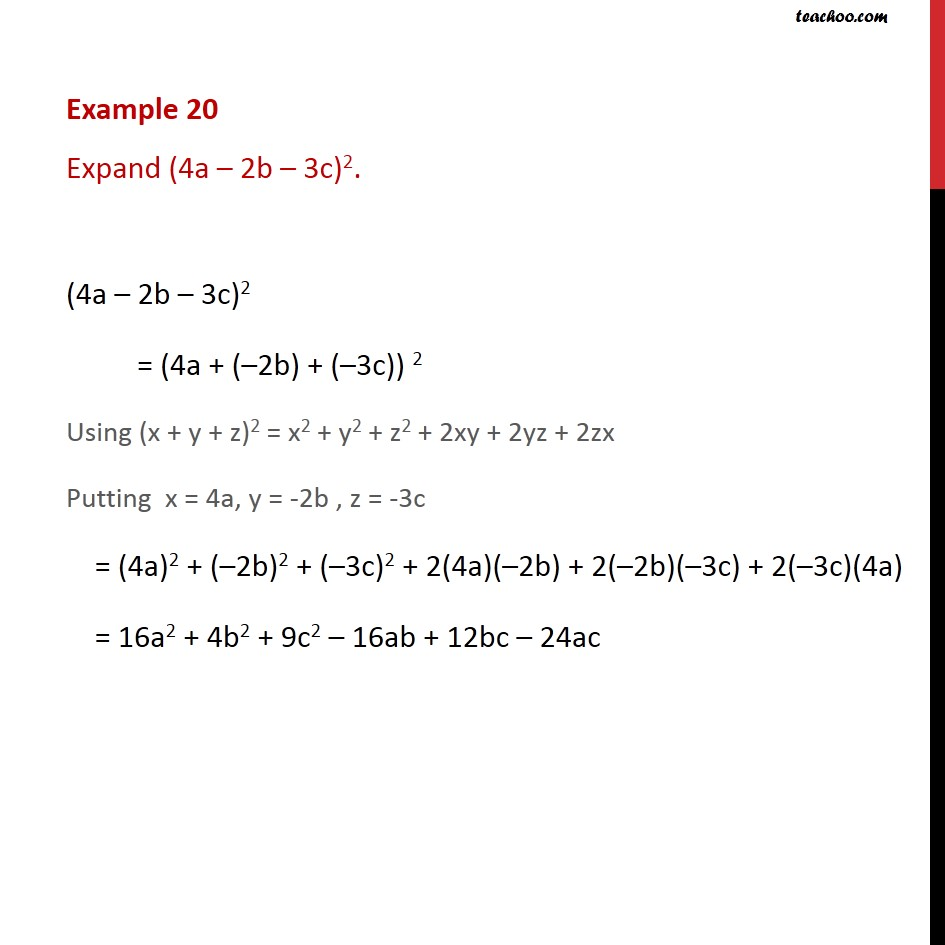Subscribe to our Youtube Channel - https://you.tube/teachoo

1. Chapter 2 Class 9 Polynomials
2. Concept wise
3. Identity V

Transcript

Example 20 Expand (4a – 2b – 3c)2. (4a – 2b – 3c)2 = (4a + (–2b) + (–3c)) 2 Using (x + y + z)2 = x2 + y2 + z2 + 2xy + 2yz + 2zx Putting x = 4a, y = -2b , z = -3c = (4a)2 + (–2b)2 + (–3c)2 + 2(4a)(–2b) + 2(–2b)(–3c) + 2(–3c)(4a) = 16a2 + 4b2 + 9c2 – 16ab + 12bc – 24ac

Identity V